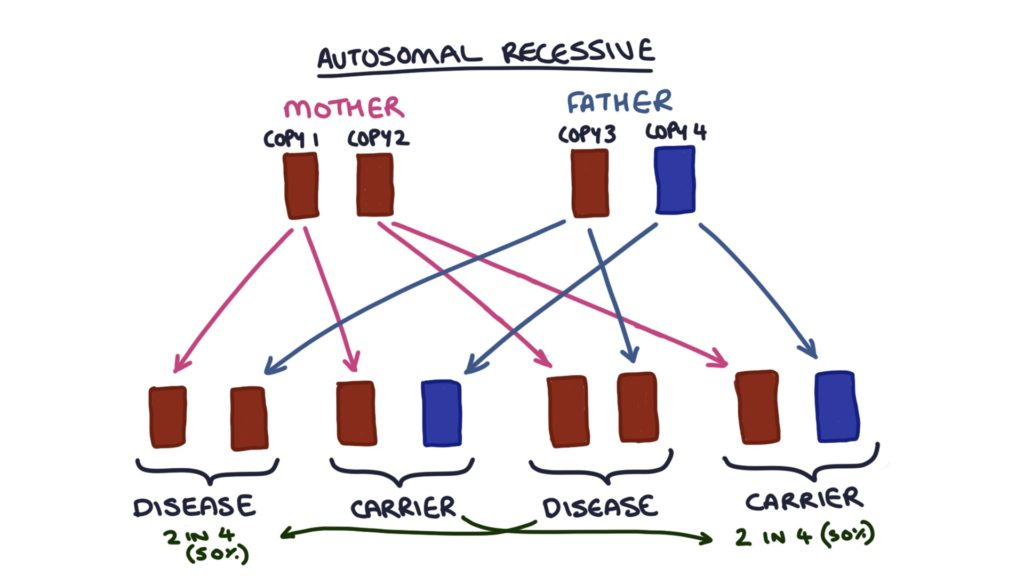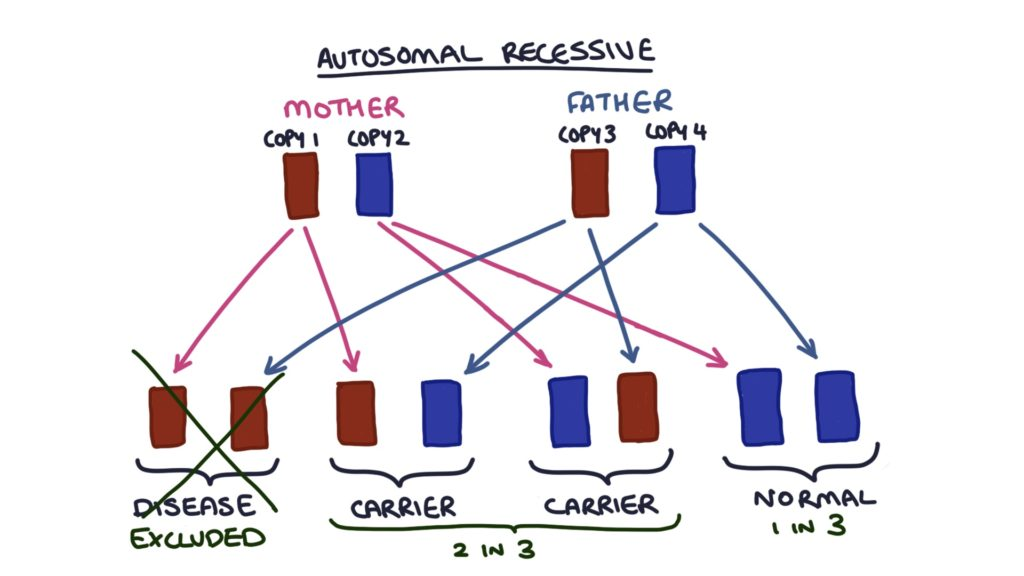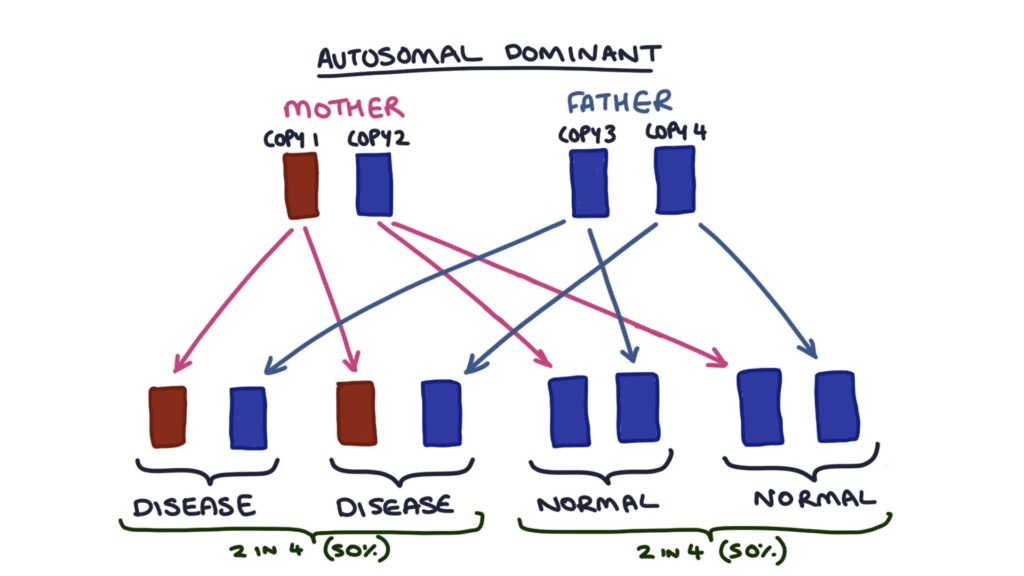# Mendelian Inheritance of Single Gene Disorders

Some genetic conditions exhibit a simple inheritance pattern called Mendelian Inheritance.

This type of inheritance only occurs where the disease is caused by a single abnormal gene on one of the non-sex chromosomes (i.e. not the X or Y chromosomes). These chromosomes are called “autosomes”.

Two examples of diseases that are caused by a single gene passed on from a patient’s parents are Huntington’s Chorea and Cystic Fibrosis. The difference between these two conditions is that the gene for Huntington’s Chorea is a dominant gene and the gene for Cystic Fibrosis is a recessive gene. This means that to have the phenotype for Huntington’s Chorea (i.e. to actually have the disease) you only need a single copy of the gene.

To have the phenotype for Cystic Fibrosis (i.e. to have cystic fibrosis) both of your copies of the gene for this disease must be the disease gene. If you only have one copy you are called a “carrier”. This means you carry the gene for the disease and can pass that on to your offspring, but you don’t have the disease itself because the other copy of the gene is normal and overrides the abnormal gene.

We refer to conditions like cystic fibrosis as “autosomal recessive” and conditions like Huntingtons as “autosomal dominant”, referring to the fact that the genes are on the non-sex chromosomes (autosomes) and that they are recessive or dominant.

### Calculating the Risk of an Autosomal Recessive Condition

It is helpful to draw out the inheritance pathway when calculating the risk of a condition. By using the following method you can always work out the risk of various outcomes based on the genetic status of the parents. To introduce this method lets look at some practical examples that you might face in your exams.

### Scenario 1

First, lets consider that both parents are carriers of an autosomal recessive condition. Each parent has one disease gene and one normal gene, and as a result they do not have the disease but do have the potential to pass on the gene for the disease to their children. Let’s label the copies of the gene that could be inherited from the parents as copy 1 – 4, with copy 1 and 2 coming from the father and copies 3 and 4 coming from the mother. There are always four possible outcomes to the inheritance of the specific gene:

• Outcome 1: Copy 1 and Copy 3
• Outcome 2: Copy 1 and Copy 4
• Outcome 3: Copy 2 and Copy 3
• Outcome 4: Copy 2 and Copy 4

Lets say Copy 1 and Copy 3 are autosomal recessive disease genes and Copy 2 and Copy 4 are normal:

• Outcome 1 has two abnormal copies and therefore has the disease.
• Outcome 2 has one abnormal copy and one normal copy and therefore becomes a carrier.
• Outcome 3 has one abnormal copy and one normal copy therefore becomes a carrier.
• Outcome 4 has two normal copies and therefore is free from the abnormal gene.Therefore the children of these parents have a 1 in 4 (or 25%) chance of having the disease, and 2 in 4 (or 50%) chance of being a carrier and a 1 in 4 (or 25%) chance of having normal genes.

### Scenario 2

Lets apply this method to another example. Lets say one parent has an autosomal recessive disease, and the other parent is a carrier. Copy 1 and Copy 2 of the gene (from the affected parent) must be abnormal, whilst in the other parent (the carrier) Copy 3 is abnormal and Copy 4 is normal. Again there are four possible outcomes to the inheritance of this gene:

• Outcome 1: Copy 1 and Copy 3
• Outcome 2: Copy 1 and Copy 4
• Outcome 3: Copy 2 and Copy 3
• Outcome 4: Copy 2 and Copy 4

Therefore:

• Outcome 1 has the disease.
• Outcome 2 is a carrier.
• Outcome 3 has the disease.
• Outcome 4 is a carrier.Therefore the children of these parents have a 50% chance of having the disease and a 50% chance of being a carrier.

You can use this method to calculate the possible outcomes in any situation.

### Scenario 3 (Popular Exam Question)

I want to illustrate one final scenario for autosomal recessive conditions that is popular in exams as it is quite good at tricking the student so pay close attention. It is usually something like this: both parents are healthy, one sibling has a single gene disease (e.g. cystic fibrosis) disease, a second child does not have the disease, what is the likelihood of the second child being a carrier. We know that the condition is autosomal recessive, because it is impossible to inherit an autosomal dominant condition without having an affected parent. Both parents must be carriers because two abnormal copies are required for the first child to have the disease and if one parent had two abnormal copies they would also have the disease. Therefore the possible outcomes in future children are the same as in the first example above:

• Outcome 1 has two abnormal copies and therefore has the condition.
• Outcome 2 has one abnormal copy and one normal copy, therefore becomes a carrier.
• Outcome 3 has one abnormal copy and one normal copy, therefore becomes a carrier.
• Outcome 4 has two normal copies and therefore is neither has the disease or is a carrier.So the risk of a future child being a carrier is 2 in 4, or 50%. However this is NOT the answer to the question as we know that the second child in the question does not have the phenotype of the disease, therefore we can exclude Outcome 1. Therefore, the possible genetic outcomes for the second child are 2, 3, or 4, and two of these outcomes (3 and 4) are that the child is a carrier. Therefore the risk of the second child being a carrier is 2 in 3.

### Calculating the Risk of an Autosomal Dominant Condition

We can use the same method for calculating the possible outcomes with autosomal dominant conditions as well. For example, lets say we want to know the possible outcomes when one parent has the condition and the other parent is disease free. We need to know whether the affected parent has one or two copies of the gene for the disease, because either way they will have the condition. We can find this out through genetic testing, or by asking about that person’s parents. If the affected person had only one affected parent, we know they only have one copy of the disease gene, however if they had two affected parents it is possible they have two copies of the disease gene. For this example, lets assume they only have one copy of the disease gene. Again, we start with four copies of the gene, two from each parent. Lets assume Copy 1 is the gene for the disease.

• Outcome 1: Copy 1 and Copy 3
• Outcome 2: Copy 1 and Copy 4
• Outcome 3: Copy 2 and Copy 3
• Outcome 4: Copy 2 and Copy 4

Therefore:

• Outcome 1 has the disease
• Outcome 2 has the disease
• Outcome 3 has two normal genes
• Outcome 4 has two normal genesTherefore the children of these parents have a 2 in 4 (or 50%) chance of having the disease, and 2 in 4 (or 50%) chance of having normal genes.